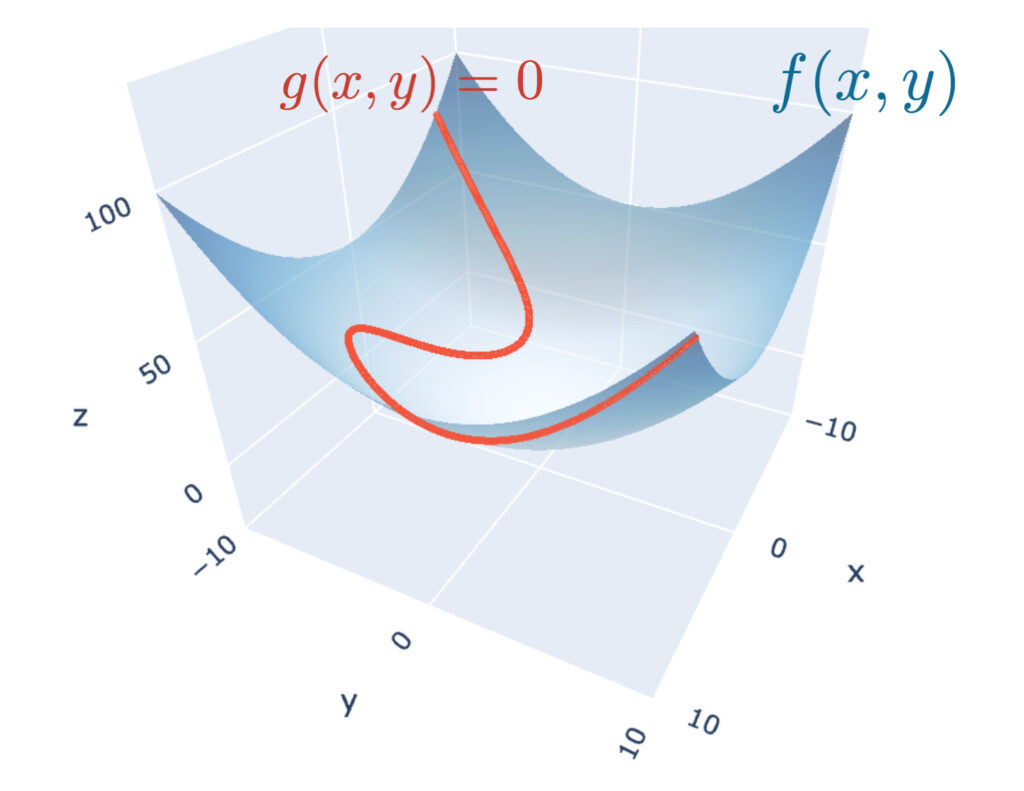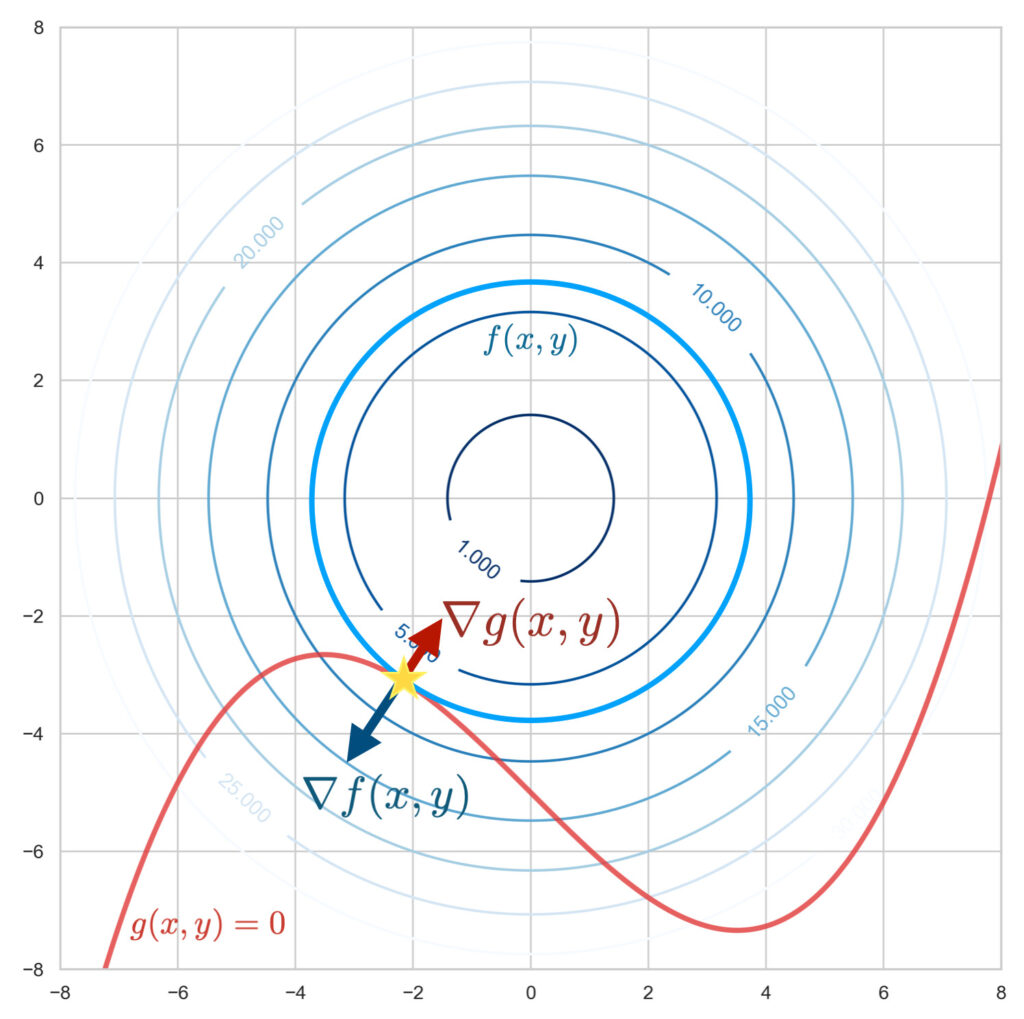# Method Of Lagrange Multiplier Under Equality Conditions

## Introduction

Mathematical optimization is the minimization (or maximization) of a function under given constraints. Such mathematical optimization problems appear in various fields such as economics and physics, and many machine learning algorithms are attributed to mathematical optimization problems. Therefore, knowing mathematical optimization and its solution methods should help us understand the fundamentals of machine learning.

This article describes Method Of Lagrange Multiplier, a powerful technique for solving mathematical optimization problems.

Minimizing a function under a constraint can be expressed as follows \begin{align*} \min_{\bm{x}}\ &f(\bm{x}), \\ {\rm subject\ to\ }\ &g(\bm{x}) = 0. \end{align*} First, let’s solve the mathematical optimization problem straightforwardly, without using Method Of Lagrange Multiplier.

As an example. \begin{align*} \min_{x,y}\ &xy, \\ {\rm subject\ to\ }\ &x – y + 4 = 0. \end{align*}

“Find the minimum value of $xy$ when the real numbers $x, y$ satisfy $x – y + 4 = 0$.”

Since $y = x + 4$ from the constraints, we have

\begin{align*} xy &= x (x + 4) \\ &= x^2 + 4x \\ &= (x + 2)^2 – 4. \end{align*}

Thus, the minimum value is $-4$ when $x=-2$.

If the number of variables is small and simple like this, it can be solved easily. But what about the following problem?

\begin{align*}
\max_{n_1, \dots, n_m}\ &\frac{N!}{\displaystyle{\prod_{i=1}^m(n_i !)}}, \\
{\rm subject\ to\ }\ &\sum_{i=1}^m n_i \varepsilon_i = E,\ \sum_{i=1}^m n_i = N. \end{align*}

Or how about the following problem?

\begin{align*}
\min_{w_1, \dots, w_n}\ &\frac{1}{2}\sqrt{w_1^2 + \cdots + w_n^2}, \\
{\rm subject\ to\ }\ &y^{(i)}\left(\sum_{j=1}^n w_j x_j^{(i)} + b\right) \geq 1, \ \ \ i = 1, 2, \cdots, n.\end{align*}

The problem seems too complex to be solved as before. （Incidentally, the former problem gives the Boltzmann distribution, which is important in physics, and the latter gives a machine learning algorithm called Support Vector Machine

\begin{align*} \newcommand{\d}{\mathrm{d}} \newcommand{\pd}{\partial} \end{align*}

For an unconstrained problem, the gradient vector of the objective function $f(\bm{x})$ is the zero vector: $\nabla_{\! \bm{x}}\,f(\bm{x}) = \bm{0}$, solving the simultaneous equations, we can find the stopping point (the point where the differentiated function is zero). If $f(\bm{x})$ is a convex function, then that point is the minimum (or maximum) value.

Is there any way to solve a problem with constraints like this one without constraints? This would make it easier to find solutions to complex problems like the above. …… Such a solution does exist! It is called the “Method Of Lagrange Multiplier”.

## Method Of Lagrange Multiplier

Method Of Lagrange Multiplier is a method for finding the stopping points of a multivariable function under constraints between variables. \begin{align*} \min_{\bm{x}}\ &f(\bm{x}), \\ {\rm subject\ to\ }\ &g(\bm{x}) = 0 \end{align*} （ Hereafter, the function we consider is a downward convex function.）

Method Of Lagrange Multiplier

We can use a real-valued function called a Lagrangian function as \begin{align*} L(\bm{x}, \lambda) = f(\bm{x}) – \lambda g(\bm{x}) \end{align*}. The problem of finding the extreme value of $f(\bm{x})$ under the constraint $g(\bm{x}) = 0$ can be attributed to the problem of finding the solution of the following simultaneous equations. \begin{align*} &\nabla_{\! \bm{x}}\, L(\bm{x}, \lambda) = \bm{0}, \\ \\ &\frac{\pd L(\bm{x}, \lambda)}{\pd \lambda} = 0. \end{align*}

In Lagrange’s undetermined multiplier method, the constrained problem can be solved as an ordinary extreme value problem by considering a Lagrangian function $L(\bm{x}, \lambda)$ that fuses the objective function $f(\bm{x})$ and the constraint formula $g(\bm{x})$. Here, the coefficients $\lambda$ of the constraint equation are called Lagrange multipliers.

As an example, let us solve the problem at the beginning of this section using Lagrange’s undetermined multiplier method.

“Find the minimum value of $xy$ when the real numbers $x, y$ satisfy $x\, – y + 4 = 0$.”

Let $f(x, y) = xy,\ g(x, y) = x\, – y + 4$.

Lagrangian function $L$ as

\begin{align*} L(x, y, \lambda) &= f(x,y)\, – \lambda g(x,y) \\ &= xy\, – \lambda(x – y + 4) \end{align*}

From Method Of Lagrange Multiplier, the extrema are $x, y$ satisfying the following simultaneous equations

\begin{align*} \frac{\pd L}{\pd x} &= y\, – \lambda = 0 \\ \frac{\pd L}{\pd y} &= x + \lambda = 0 \\ \frac{\pd L}{\pd \lambda} &= -x + y -4 = 0 \end{align*}

Solving the above simultaneous equations, we obtain $x = -2, y = 2, \lambda = 2$.

Thus, the minimum value is $-4$.

Lagrange’s undetermined multiplier method also appears in the theory of principal component analysis in machine learning algorithms.

Principal Component Analysis (PCA) Theory
The theory of principal component analysis (PCA), a method of dimensional compression, is explained. Principal Component Analysis is a method to summarize the information of multidimensional data observed on mutually correlated features into new features expressed as a linear combination of the original features without losing any information as much as possible.

## Why is it established?

We will examine the geometry of the two-variable function case without rigor to see why it is valid. The objective function $f(x, y)$ and the constraint function $g(x, y) = 0$ are illustrated in the figure; the two-variable function $f(x, y)$ is drawn as a surface and $g(x, y) = 0$ as a curve.

Finding the minimum of the objective function under the constraints means finding the lowest elevation of the objective function: $f(x, y)$ on this curve: $g(x, y) = 0$.▲The objective function $f(x, y)$ is represented by a blue surface and the constraint function $g(x, y)=0$ by a red curve.

Suppose this curve is a mountain road. When you are at the lowest elevation along the road, you should be walking along the contour line. Because if you are going up or down a mountain road, you are going across the contour line. The moment of lowest elevation along the road is the position where you are not crossing the contour line, i.e., you are walking along the contour line. This can be regarded as a geometric situation where the tangent line of the curve overlaps the tangent line of the contour line.▲The objective function $f(x,y)$ is shown with contour lines. The lowest elevation on the red curve ($g(x,y)=0$) is where the tangent line of the curve and the contour line overlap (the starred point in the figure). This is also the point where the gradient vectors of the two functions are parallel.

To find the point where these two tangents overlap (indicated by the star in the figure above), we can use the gradient of the function. The gradient vector $\nabla_{\! x, y}\, f(x, y),\ \nabla_{\! x, y}\, g(x, y)$ are vectors perpendicular to the contour and curve, respectively.

The gradient $\nabla_{\! x, y}$ and the gradient $\nabla_{\! x, y}$ are parallel is the point where the two tangents overlap, i.e., where the objective function is minimized under the constraints. This can be expressed by the following equation. \begin{align*} \nabla_{\! x, y}\, f(x,y) = \lambda \nabla_{\! x, y}\, g(x, y) \end{align*}Here, $\lambda$ is a variable that appears because the gradient directions of $f$ and $g$ need only coincide and their lengths do not matter, and is called the “Lagrange multiplier”.

By transforming the above equation, we obtain \begin{align*} \nabla_{\! x, y}\, \left\{f(x,y) – \lambda g(x, y) \right\} = 0 \end{align*}Using the Lagrangian function: $L(x, y) \equiv f(x, y) – \lambda g(x, y)$, It can be rewritten as \begin{align*} \nabla_{\! x,y}\, L(x, y) = 0 \end{align*}.

On the other hand, the constraint $g(x, y) = 0$ can be expressed as $\frac{\pd L}{\pd \lambda} = 0$.

Thus, Method Of Lagrange Multiplier allows us to solve constrained problems as ordinary extreme value problems.Yukkuri Machine Learning
タイトルとURLをコピーしました